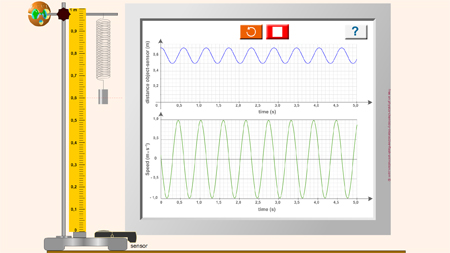VERTICAL OSCILLATOR

# VERTICAL OSCILLATOR

Description: Interactive free flash animation to simulate an activity to find the relationship between the period T and the mass m of the object.

Instructions:

1. Find k and l0:

Measure the length l of the spring for two different values of the mass m of the object.

Having put both pairs (m ; l) in the right equations, calculate the values of the spring constant k and of the length l0 relative to the equilibrium position.

2. Does the period T of oscillations depend on the mass m of the object?

For eight different values from the mass of the object, activate oscillations of the system {mass + spring} by taking away the object of its equilibrium position and by releasing it.

- Measure the oscillations period T for each value of the mass m.

- Calculate T2 for each value of the period T.

- Note the pairs of values (T2 ; m) into an array.

- Plot the curve of T2 = f(m)

a. Does the period T of oscillations depend on the mass m of the object?

b. What would be the period of the oscillations of an object with the mass of m = 88 g?
Show the method used by drawing on the graph.

c. What is the nature of the given curve?
Determine its gradient expressed in s².kg-1.

d. Deduct from it the relationship between T2 and m.

Definition:

Simple pendulum: Hypothetical pendulum suspended by a massless and frictionless thread of constant length.

Video: PCCL does not post a video here as it is up to the students to complete the activity.